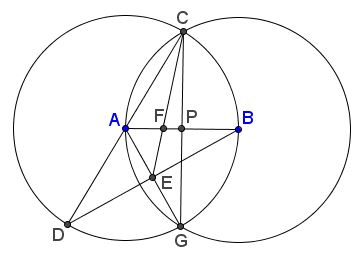# Trisect Segment: 2 Circles, 4 Lines

The diagram below illustrates trisections of a given segment $(AB)$ that requires drawing two circles and four lines:### Proof

Observe that, by the construction, in the diagram below, $P$ is the midpoint of $CG$ while $E$ is the midpoint of $AG.$ It thus follows that $F$ is the centroid in $\Delta ACG,$ implying $AF:FP=2:1,$ but $P$ is also the midpoint of $AB.$ We therefore get the sequence of ratios: $AP:AF=3:2,$ $AB:AF=6:2=3:1.$In the article referred to below a similar (but even shorter) argument was applied to $\Delta BCD,$ with $CE$ and $AB$ as the medians.

### References### Various Geometric Constructions

• How to Construct Tangents from a Point to a Circle
• How to Construct a Radical Axis
• Constructions Related To An Inaccessible Point
• Inscribing a regular pentagon in a circle - and proving it
• The Many Ways to Construct a Triangle and additional triangle facts
• Easy Construction of Bicentric Quadrilateral
• Easy Construction of Bicentric Quadrilateral II
• Star Construction of Shapes of Constant Width
• Four Construction Problems
• Geometric Construction with the Compass Alone
• Construction of n-gon from the midpoints of its sides
• Short Construction of the Geometric Mean
• Construction of a Polygon from Rotations and their Centers
• Squares Inscribed In a Triangle I
• Construction of a Cyclic Quadrilateral
• Circle of Apollonius
• Six Circles with Concurrent Pairwise Radical Axes
• Tangent to Circle in Three Steps
• Regular Pentagon Construction by K. Knop
•